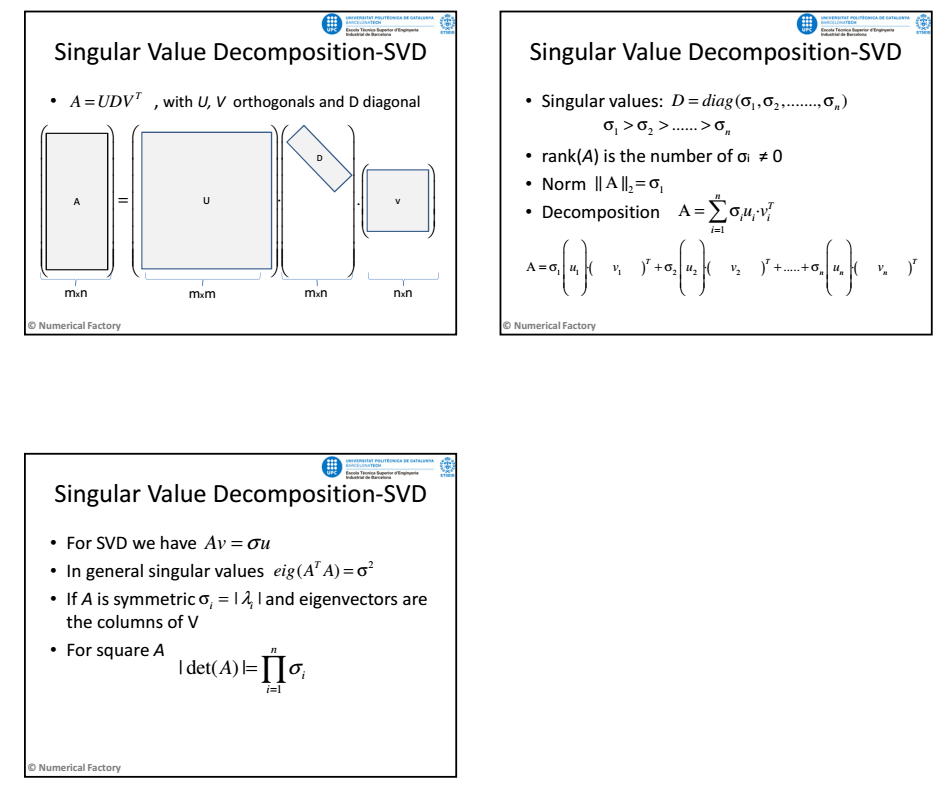# SVD Decomposition: Usage on the comprehension of images

Given a mxn matrix, its SVD decomposition (Singular Value Decomposition) gives a change of basis in which the matrix is a diagonal matrix. The basis of this process is orthonormal and the values of the diagonal (singular values) give information about the original matrix.
This process is normally used for SVD while solving lineal equation systems as Ax=b## SVD in Matlab

The function which let's you obtain the SVD decomposition on Matlab is svd
A=ones(3,4)+10*eye(3,4) %We create a NONE square matrix to be able to see the different dimensions
A = 3×4
11 1 1 1 1 11 1 1 1 1 11 1
[U,D,V]=svd(A)
U = 3×3
0.5774 0 0.8165 0.5774 0.7071 -0.4082 0.5774 -0.7071 -0.4082
D = 3×4
13.1149 0 0 0 0 10.0000 0 0 0 0 10.0000 0
V = 4×4
0.5723 0.0000 0.8165 -0.0762 0.5723 0.7071 -0.4082 -0.0762 0.5723 -0.7071 -0.4082 -0.0762 0.1321 -0.0000 0.0000 0.9912
A-U*D*V' %see that the V matrix must be transposed (is the invers!) to be able to change the basis.
ans = 3×4
10-14 ×
0 0.0111 -0.0222 0 -0.3109 0.3553 0.0888 0.0111 -0.2887 0.0888 0.1776 0.0111
S=diag(D) %Singular values
S = 3×1
13.1149 10.0000 10.0000
Note that U and V matrix are orthogonal
U*U' %The product between the U vectors
ans = 3×3
1.0000 -0.0000 -0.0000 -0.0000 1.0000 -0.0000 -0.0000 -0.0000 1.0000
V*V' %the product between the V vectors
ans = 4×4
1.0000 0.0000 0.0000 0.0000 0.0000 1.0000 -0.0000 -0.0000 0.0000 -0.0000 1.0000 -0.0000 0.0000 -0.0000 -0.0000 1.0000
The matrix range is the number of singular values that differ from zero
ind=find(S > 0); %only needed the > 0,because all the singular values are positive
rang=length(ind); %The length of the vector gives the total number
% we compare with the Matlab function that returns the range of a matrix (rank(A))
[rang, rang-rank(A)] %we show the value and the checking
ans = 1×2
3 0

## Matrix succesive approximation:

The SVD decomposition allows approaching a matrix successively, that way the error is of the same order of the first used singular value
A1=A-S(1)*U(:,1)*V(:,1)' %approximation with the frist singular value
A1 = 3×4
6.6667 -3.3333 -3.3333 0.0000 -3.3333 6.6667 -3.3333 -0.0000 -3.3333 -3.3333 6.6667 0
A2=A-(S(1)*U(:,1)*V(:,1)'+S(2)*U(:,2)*V(:,2)') %first and second
A2 = 3×4
6.6667 -3.3333 -3.3333 0.0000 -3.3333 1.6667 1.6667 -0.0000 -3.3333 1.6667 1.6667 0
A3=A-(S(1)*U(:,1)*V(:,1)'+S(2)*U(:,2)*V(:,2)'+S(3)*U(:,3)*V(:,3)') %all
A3 = 3×4
10-14 ×
0 0 -0.0444 0 -0.2665 0.5329 0.0888 0 -0.3109 0.1110 0.1776 0.0222
[norm(A1),norm(A2),norm(A3)]
ans = 1×3
10.0000 10.0000 0.0000

## Application: Image compression

If we consider that an image is a numeric matrix, the SVD decomposition can be used to approach this image using only a few singular values of this image, reducing the values that need to be saved to approach the image (compression)